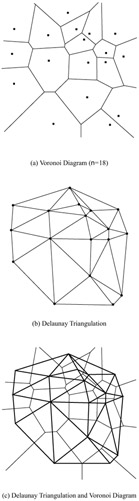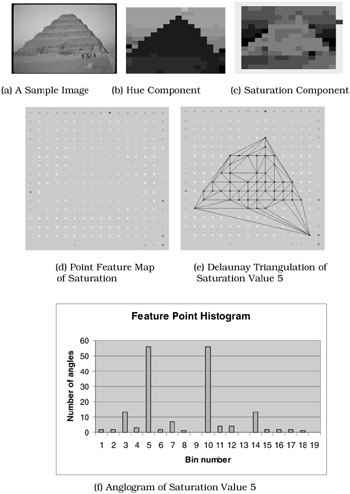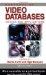# 3. Color Anglogram

## 3. Color Anglogram

In this section, we will introduce our color anglogram approach, which is a spatial color indexing technique based on Delaunay triangulation. We will first give some Delaunay triangulation-related concepts in computational geometry, and then present the geometric triangulation-based anglogram representation for encoding spatial correlation, which is translation, scale, and rotation invariant.

Let P = {p1, p2, ..., pn} be a set of points in the two-dimensional Euclidean plane, namely the sites. Partition the plane by labeling each point in the plane to its nearest site. All those points labeled as pi form the Voronoi region V(pi). V(pi) consists of all the points x's at least as close to pi as to any other site:

V(pi) = {x:|pi - x| | pj - x|, j i}

Some points x's do not have a unique nearest site. The set of all points that have more than one nearest site form the Voronoi diagram V(P) for the set of sites.

Construct the dual graph G for a Voronoi Diagram V(P) as follows: the nodes of G are the sites of V(P), and two nodes are connected by an arc if their corresponding Voronoi polygons share a Voronoi edge. In 1934, Delaunay proved that when the dual graph is drawn with straight lines, it produces a planar triangulation of the Voronoi sites P, so called the Delaunay triangulation D(P). Each face of D(P) is a triangle, so called the Delaunay triangle.

For example, Figure 15.1 (a) shows the Voronoi diagram for a number of 18 sites. Figure 15.1(b) shows the corresponding Delaunay triangulation for the sites shown in Figure 15.1(a), and Figure 15.1(c) shows the Voronoi diagram in Figure 15.1 (a) superimposed on the corresponding Delaunay triangulation in Figure 15.1(b). We note that it is not immediately obvious that using straight lines in the dual would avoid crossings in the dual. The dual segment between two sites does not necessarily cross the Voronoi edge shared between their Voronoi regions, as illustrated in Figure 15.1(c).Figure 15.1: A Delaunay Triangulation Example

The proof of Delaunay's theorems and properties is beyond the scope of this chapter, but can be found in . Among various algorithms for constructing the Delaunay triangulation of a set of N points, we note that there are O(NlogN) algorithms [11, 15] for solving this problem.

Spatial layout of a set of points can be coded through such an anglogram that is computed by discretizing and counting the angles produced by the Delaunay triangulation of a set of unique feature points in the context, given the selection criteria of what the bin size will be, and of which angles will contribute to the final angle histogram. An important property of our proposed anglogram for encoding spatial correlation is its invariance to translation, scale, and rotation. An O(max(N, #bins)) algorithm is necessary to compute the anglogram corresponding to the Delaunay triangulation of a set of N points.

The color anglogram technique is based on the Delaunay triangulation computed on visual features of images. To construct color anglograms, color features and their spatial relationship are extracted and then coded into the Delaunay triangulation. Each image is decomposed into a number of non-overlapping blocks. Each individual block is abstracted as a unique feature point labeled with its spatial location and feature values. The feature values in our experiment are dominant or average hue and saturation in the corresponding block. Then, all the normalized feature points form a point feature map for the corresponding image. For each set of feature points labeled with a particular feature value, the Delaunay triangulation is constructed and then the feature point histogram is computed by discretizing and counting the number of either the two large angles or the two small angles in the Delaunay triangles. Finally, the image will be indexed by using the concatenated feature point histogram for each feature value. Figure 15.2(a) shows a pyramid image of size 192 128. By dividing the image into 256 blocks, Figure 15.2(b) and Figure 15.2(c) show the image approximation using dominant hue and saturation values to represent each block, respectively. Figure 15.2(d) presents the corresponding point feature map perceptually. Figure 15.2(e) is the Delaunay triangulation of the set of feature points labeled with saturation value 5, and Figure 15.2(f) shows the corresponding anglogram obtained by counting the two largest angles of each triangle. A sample query with color anglogram is shown in Figure 15.3.Figure 15.2: A Color Anglogram ExampleFigure 15.3: A Sample Query Result of Color AnglogramHandbook of Video Databases: Design and Applications (Internet and Communications)
ISBN: 084937006X
EAN: 2147483647
Year: 2003
Pages: 393